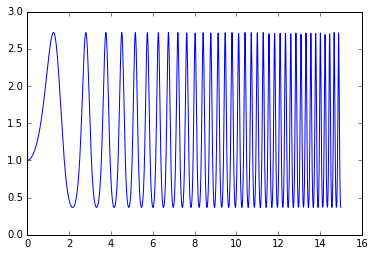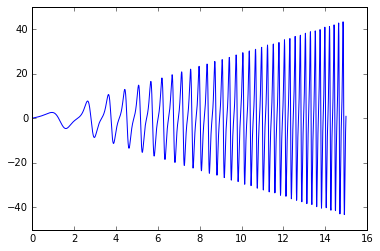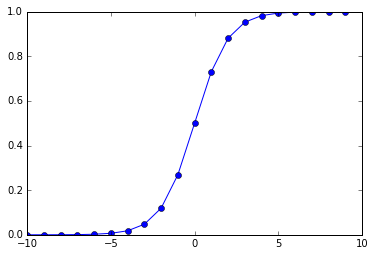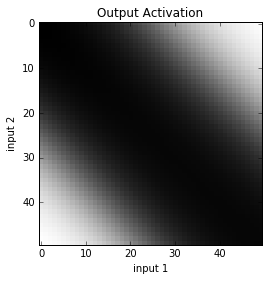# Introduction to Theano¶

What is Theano? Theano is a method of describing mathematical operations that can be carried out either in Python or by low-level C code on a Graphics Processing Unit (GPU).

## Simple Example¶

To get started, let's explore a very simple example. Consider the function:

$f(x, y) = x + y$

In mathematics, we write the function $f$ that takes two parameters, $x$ and $y$. The body of the function is simply $x + y$.

In Python, we would write that as:

In :
def f(x, y):
return x + y

In :
f(5, 6)

Out:
11

In Theano, we use the Python language as a method of writing symbolic expressions.

First, we import the needed components T and function:

In :
import theano.tensor as T
from theano import function


Next, we define two symbols, x and y. These are both Python variables and Theano symbols. The quoted 'x' is the Theano symbol, which is assigned to a Python variable of the same name (to make it easier to understand and help during debugging).

In :
x = T.scalar('x')


The term scalar simply means that it has a single value. In Theano, this is sometimes called a zero-dimensional array.

We do the same with y:

In :
y = T.scalar('y')

# or both together:
x, y = T.scalars('x', 'y')


As you can see, the Python variable x is of type TensorVariable.

In :
type(x)

Out:
theano.tensor.var.TensorVariable

Next, we define a Theano function. We do this in two steps. First, we describe the body of the function:

In :
func = x + y


This looks like we are adding x and y together. However, we note that x and y are symbols... they do not yet have values.

We can see that func is something very strange:

In :
func

Out:
Elemwise{add,no_inplace}.0

The second step in creating a function is to make a Python function. To do this, we use the Theano function function. It takes a list of parameters as symbols, followed by the Theano function body:

In :
pyfunc = function(inputs=[x, y], outputs=func)
# or:
# pyfunc = function([x, y], func)

In :
pyfunc

Out:
<theano.compile.function_module.Function at 0x7fe7e61e0a20>
In :
pyfunc(5, 6)

Out:
array(11.0)

You can take a Theano function body and show it in symbolic form, perhaps close to the original Python source:

In :
from theano import pp
pp(func)

Out:
'(x + y)'

## Matrix Example¶

This example will use matrices, one of the main uses of Theano.

In this example, we will use T.dmatrix to define a matrix of doubles (floating point values).

In :
a = T.dmatrix('a')
b = T.dmatrix('b')


Again, we define the Theano function, and the Python function:

In :
func = a + b
pyfunc = function([a, b], func)

In :
pyfunc([[1, 2],
[3, 4]],
[[10, 20],
[100, 200]])

Out:
array([[  11.,   22.],
[ 103.,  204.]])

The result is given as a numpy ndarray:

In :
result = pyfunc([[1, 2],
[3, 4]],
[[10, 20],
[100, 200]])

In :
type(result)

Out:
numpy.ndarray

You can create matrices composed of:

• 32-bit itegers (i prefix)
• 64-bit integers (l prefix)
• 32-bit floats (f prefix)
• 64-bit floats (d prefix)

## Computing a Derivative¶

Consider the equation:

$f(x)=e^{sin(x^2)}$

In :
x = T.dscalar('x')

In :
fx = T.exp(T.sin(x**2))

In :
pyfunc = function([x], fx)

In :
pyfunc(10)

Out:
array(0.602681965908778)

Does that agree with what we would compute directly in Python?

In :
import math
def eq(x):
return math.exp(math.sin(x ** 2))
eq(10)

Out:
0.602681965908778

Yes, they do agree.

Let's plot the function using matplotlib.

In :
%matplotlib inline
from matplotlib.pyplot import plot

In :
import numpy
plot(numpy.arange(0, 15, .01),
[pyfunc(x) for x in numpy.arange(0, 15, .01)])

Out:
[<matplotlib.lines.Line2D at 0x7fe7e6f027b8>]Now we will compute the derivative of our function. Normally, in regular Python, we would have to solve for the derivative. However, because we have described the function symbolically, Theano can solve for the derivative via the symbols.

To do this, we will use T.grad() with respect to (wrt) x:

In :
fp = T.grad(fx, wrt=x)
fprime = function([x], fp)

In :
fprime(10)

Out:
array(10.394080663811636)

Let's plot the derivative of the function:

In :
import numpy
plot(numpy.arange(0, 15, .01),
[fprime(x) for x in numpy.arange(0, 15, .01)])

Out:
[<matplotlib.lines.Line2D at 0x7fe7e5f1f390>]## Neural Network: XOR¶

For this experiment, we will learn the function XOR using back-propagation of error.

First, we import the elements from theano and numpy that we will need:

In :
import theano
import theano.tensor as T
from theano import function, pp
import theano.tensor.nnet as nnet
import numpy as np
import random

In :
%matplotlib inline
from matplotlib.pyplot import plot


Recall XOR:

Input 1 Input 2 Target
0 0 0
0 1 1
1 0 1
1 1 0

That is, given 2 inputs the output is True if either of the inputs is True, but not if both are.

Three layers:

• 2 inputs (+ 1 bias = 3 values)
• 2 hidden units (+ 1 bias = 3 values)
• 1 output unit (1 value)

First we define two Theano symbols for representing the inputs and desired output (called the target).

In :
th_inputs = T.dvector('inputs') # two inputs
th_target = T.dscalar('target') # one target/output


To compute the activation at a layer, we take the inputs * weights to get the net activation, and then apply the sigmoid:

In :
def compute_activation(inputs, weights):
bias = np.array(, dtype='float64')
all_inputs = T.concatenate([inputs, bias])
net_input = T.dot(weights.T, all_inputs)
activation = nnet.sigmoid(net_input)
return activation


To give you a sense of what compute_activation is, you can see a representation of the computation:

In :
pp(compute_activation(th_inputs, th_target))

Out:
'sigmoid((target * join(TensorConstant{0}, inputs, TensorConstant{(1,) of 1.0})))'

What does the sigmoid function return? Let's turn that into a Python function and plot it:

In :
x = T.dscalar('x')          # 64-bit float
fx = nnet.sigmoid(x)        # Theano function

In :
fx

Out:
sigmoid.0
In :
pp(fx)

Out:
'sigmoid(x)'
In :
sigmoid = function([x], fx) # Python function

In :
sigmoid(.5)

Out:
array(0.6224593312018546)
In :
xs = range(-10, 10, 1)
ys = [sigmoid(x) for x in xs]
plot(xs, ys, "o-")

Out:
[<matplotlib.lines.Line2D at 0x7fae42bfd2e8>]We create a shared variable named epsilon to control the learning rate. The learning rate will typically be in the range 0.9 to 0.01. This value depends on the function being learned.

In :
epsilon = theano.shared(0.1, name='epsilon') # learning rate


Now we define the method to update the weights. We find the derivative with respect to the weights, and subtract that value from the weights.

In :
def compute_delta_weights(compute_error, weights):
return weights - (epsilon * T.grad(compute_error, wrt=weights))


We define the first set of weights to go between the inputs and the hidden layer.

In :
NUM_INPUTS = 2
NUM_HIDDENS = 2
NUM_OUTPUTS = 1


The initial random weights are created to span from -1 to 1.

In :
def make_weights(ins, outs):
return np.array(2 * np.random.rand(ins + 1, outs) - 1,
dtype='float64')

In :
weights1 = theano.shared(make_weights(NUM_INPUTS, NUM_HIDDENS),
name='weights1')

In :
hidden_layer = compute_activation(th_inputs, weights1)


We can test the hidden_layer Theano function by turning it into a Python function, and calling it with [0, 0] bound to th_inputs:

In :
function([th_inputs], hidden_layer)([0, 0])

Out:
array([ 0.70860966,  0.59814888])
In :
weights2 = theano.shared(make_weights(NUM_HIDDENS, NUM_OUTPUTS),
name='weights2') # 4 x 1

In :
output_layer = T.sum(compute_activation(hidden_layer, weights2)) # Theano function

In :
compute_error = (output_layer - th_target) ** 2  # Theano function


We can test the entire Theano equation now by calling compute_error with values for th_inputs and th_target:

In :
function([th_inputs, th_target], compute_error)([0, 0], 0)

Out:
array(0.1820956722056817)

Finally, we can create a Python function (called train) that will call compute_error, and update the weights:

In :
train = function(
inputs=[th_inputs, th_target],
outputs=compute_error,
(weights2, compute_delta_weights(compute_error, weights2))])


Ok, now we are ready to train a neural network to perform the XOR function.

In :
inputs = [[0, 1],
[1, 0],
[1, 1],
[0, 0]]

def xor(a, b):
return int((a or b) and not(a and b))

for pattern in inputs:
print(pattern, xor(*pattern))

[0, 1] 1
[1, 0] 1
[1, 1] 0
[0, 0] 0


We simply call train() on each of the input/target pairs:

In :
def train_all(epochs=5000):
for e in range(epochs):
random.shuffle(inputs)
for i in range(len(inputs)):
target = xor(*inputs[i])
error = train(inputs[i], target)
if (e + 1) % 500 == 0 or e == 0:
print('Epoch:', e + 1, 'error:', error)

In :
%%time
train_all()

Epoch: 1 error: 0.17590230024029582
Epoch: 500 error: 0.21345970272465195
Epoch: 1000 error: 0.12049236516936544
Epoch: 1500 error: 0.058610122233544594
Epoch: 2000 error: 0.021417091129154138
Epoch: 2500 error: 0.009440614304426448
Epoch: 3000 error: 0.00704809674144815
Epoch: 3500 error: 0.0040809552182693035
Epoch: 4000 error: 0.0026386841665036777
Epoch: 4500 error: 0.0027368789131071805
Epoch: 5000 error: 0.002107508288249151
CPU times: user 3.96 s, sys: 0 ns, total: 3.96 s
Wall time: 3.96 s

In :
test = function([th_inputs], output_layer)

In :
test([0, 0])

Out:
array(0.04786447075736276)
In :
test([0, 1])

Out:
array(0.9468603709518669)
In :
test([1, 0])

Out:
array(0.9541607426531297)
In :
test([1, 1])

Out:
array(0.04242692545605788)

To train another network, we need to reinitialize the weights:

In :
NUM_HIDDENS = 5

In :
weights1.set_value(make_weights(NUM_INPUTS, NUM_HIDDENS))
weights2.set_value(make_weights(NUM_HIDDENS, NUM_OUTPUTS))


We could also try a different learning rate:

In :
epsilon.set_value(0.05)

In :
%%time
train_all()

Epoch: 1 error: 0.5129482764713498
Epoch: 500 error: 0.27343068436219553
Epoch: 1000 error: 0.26608887763525896
Epoch: 1500 error: 0.23315802349319936
Epoch: 2000 error: 0.27206627125525334
Epoch: 2500 error: 0.14440235701111373
Epoch: 3000 error: 0.06289470998854373
Epoch: 3500 error: 0.032000729577620186
Epoch: 4000 error: 0.02204097166832901
Epoch: 4500 error: 0.014695800522454913
Epoch: 5000 error: 0.010053228953848652
CPU times: user 3.96 s, sys: 0 ns, total: 3.96 s
Wall time: 3.97 s

In :
for pattern in inputs:
print(pattern, test(pattern))

[0, 0] 0.08647435393170991
[1, 1] 0.10474032896915239
[0, 1] 0.89557766834058
[1, 0] 0.9001455909520547


## Generalization¶

Although we only trained on the corners, we can see what

In :
import matplotlib.pyplot as plt

res = 50 # resolution
z = np.zeros((res, res))

for x in range(res):
for y in range(res):
z[x][y] = test([x/res, y/res])

plt.imshow(z, cmap=plt.cm.gray, interpolation='nearest')
plt.xlabel("input 1")
plt.ylabel("input 2")
plt.title("Output Activation")

plt.show()# Handwriting Categorization¶

First, I'll use the metakernel's %download magic to get the MNIST hand written data:

In :
! pip install metakernel --user -U

Requirement already up-to-date: metakernel in /home/dblank/.local/lib/python3.4/site-packages
Requirement already up-to-date: IPython>=3.0 in /usr/local/lib/python3.4/dist-packages (from metakernel)
Requirement already up-to-date: prompt-toolkit<2.0.0,>=1.0.3 in /usr/local/lib/python3.4/dist-packages (from IPython>=3.0->metakernel)
Requirement already up-to-date: pygments in /usr/local/lib/python3.4/dist-packages (from IPython>=3.0->metakernel)
Requirement already up-to-date: pickleshare in /usr/local/lib/python3.4/dist-packages (from IPython>=3.0->metakernel)
Requirement already up-to-date: simplegeneric>0.8 in /usr/lib/python3/dist-packages (from IPython>=3.0->metakernel)
Requirement already up-to-date: traitlets>=4.2 in /usr/local/lib/python3.4/dist-packages (from IPython>=3.0->metakernel)
Requirement already up-to-date: setuptools>=18.5 in /usr/local/lib/python3.4/dist-packages (from IPython>=3.0->metakernel)
Requirement already up-to-date: pexpect; sys_platform != "win32" in /usr/local/lib/python3.4/dist-packages (from IPython>=3.0->metakernel)
Requirement already up-to-date: decorator in /usr/local/lib/python3.4/dist-packages (from IPython>=3.0->metakernel)
Requirement already up-to-date: wcwidth in /usr/local/lib/python3.4/dist-packages (from prompt-toolkit<2.0.0,>=1.0.3->IPython>=3.0->metakernel)
Requirement already up-to-date: six>=1.9.0 in /usr/local/lib/python3.4/dist-packages (from prompt-toolkit<2.0.0,>=1.0.3->IPython>=3.0->metakernel)
Requirement already up-to-date: ipython-genutils in /usr/local/lib/python3.4/dist-packages (from traitlets>=4.2->IPython>=3.0->metakernel)
Requirement already up-to-date: ptyprocess>=0.5 in /usr/local/lib/python3.4/dist-packages (from pexpect; sys_platform != "win32"->IPython>=3.0->metakernel)

In :
import metakernel

In :
metakernel.register_ipython_magics()

In :
%download http://deeplearning.net/data/mnist/mnist.pkl.gz

Downloaded 'mnist.pkl.gz'.


Unzip the file:

In :
!gunzip mnist.pkl.gz


From http://deeplearning.net/tutorial/gettingstarted.html we see that:

The pickled file represents a tuple of 3 lists: the training set, the validation set and the testing set. Each of the three lists is a pair formed from a list of images and a list of class labels for each of the images. An image is represented as numpy 1-dimensional array of 784 (28 x 28) float values between 0 and 1 (0 stands for black, 1 for white). The labels are numbers between 0 and 9 indicating which digit the image represents.

We read the Python2 pickled data:

In :
import pickle
import gzip
import numpy

with open('mnist.pkl', 'rb') as f:
u = pickle._Unpickler(f)
u.encoding = 'latin1'
train_set, validation_set, test_set = data

In :
len(train_set)

Out:
2
In :
len(train_set)

Out:
50000
In :
len(train_set)

Out:
784
In :
epsilon.set_value(0.1)

In :
weights1.set_value(make_weights(784, 100))
weights2.set_value(make_weights(100, 1))

In :
inputs = [train_set[i] for i in range(len(train_set))]
targets = [train_set[i]/9.0 for i in range(len(train_set))]

In :
def display_digit(vector):
for r in range(28):
for c in range(28):
v = int(vector[r * 28 + c] * 10)
ch = " .23456789"[v]
print(ch, end="")
print()

In :
for i in range(10):
display_digit(inputs[i])
print(int(targets[i] * 9))



456.6994
..36699999869972
.99999999993332.
8999997799
364998  .6
693
597
792
.9864
39994
.7995.
3997
9992
.57998
.5899997
48999973
28999973
68999973
268999995
5999855

5

.696.
.99999
28999992
2899973994
69999993796
.9997498.396
.9996 24   99.
.69983      996
6992 .      997
2992         997
797          997
2994          995
398          597
398         5982
395       .696
398      4996
3995..3689862
39998899975
.79999995
59995.

0

29.
23              47.
46              58.
86             .96
86             794
.96             792
496             99.
694            698
692          36993
693   ..455999699.
5998889999753. 99
46666632     398
695
692
692
693
693
695
695
395

4

4992
39992
49992
2998.
28993
6997
9992
.8994
4997
3997
.9996
5999.
.8996
9997
9993
6999.
.8995
2998
2998
798

1

258994352
3999789996
29972  7994
3997   3998
5995    8993
4996    3995
996   .7996
99. 4799993
9999998899
29985. 795
2994
299
99
299
499
.99
892
397.
796
59.

9

34
.589995
.59998899.
599998..992
399998. .992
79986.  3992
.52     3992
6992
4444797
26999998.
28998699994
39942  899993
5997   799569972..
5994  27982  269998
4993 26994.     377.
999889994
8999964.
354

2

598.
.9992
6992
5992
7992
.8994.
49994
9994
9994
9994
9994
9994
9996
9999.
59995
49995
8999.
6999.
2999.
.899.

1

..49999996
.588999999996
69999999999992
49985555799992
..      8996
39995
37999
3899997
.4777999993.
.8999999996
.89999999992
.42....5992
992
3992
2       39992
279.     399992
89955555799973
899999999985
.699999885.
49964

3

27
98
99.
99.
992
994
995
695
695
696
699
499
399.
399.
895
59
59
593
89.
68.

1

77
595
5993
797
27     398.
892    893
892   .993
598    697
.992   598
.895   4994
399974  796
39998997899
39992.7999998.
783   .799996
4.    2993..
598
499.
.895
896
493

4

In :
def train_digits(max_epochs=1000):
epoch = 0
correct = 0
total = 1
while correct/total < 1.0 and epoch < max_epochs:
total = 0
correct = 0
for i in range(100):
target = targets[i]
error = train(inputs[i], target)
output = test(inputs[i])
target = int(targets[i] * 9)
total += 1
if int(output * 10) == target:
correct += 1
if (epoch + 1) % 10 == 0 or epoch == 0:
print('Epoch:', epoch + 1, 'error:', error, "% correct:", correct/total)
epoch += 1

In :
train_digits()

Epoch: 1 error: 0.005584251108050222 % correct: 0.35
Epoch: 10 error: 0.0029438254302019415 % correct: 0.63
Epoch: 20 error: 0.006812425881126288 % correct: 0.82
Epoch: 30 error: 0.007693440417472783 % correct: 0.86
Epoch: 40 error: 0.007743773335648142 % correct: 0.87
Epoch: 50 error: 0.007499984180644583 % correct: 0.87
Epoch: 60 error: 0.006956871273104968 % correct: 0.88
Epoch: 70 error: 0.006286300074310477 % correct: 0.91
Epoch: 80 error: 0.005599327283432899 % correct: 0.94
Epoch: 90 error: 0.004935531392142657 % correct: 0.94
Epoch: 100 error: 0.004342748344285977 % correct: 0.94
Epoch: 110 error: 0.003883171178634236 % correct: 0.95
Epoch: 120 error: 0.0035272259137190546 % correct: 0.96
Epoch: 130 error: 0.003215317224888725 % correct: 0.96
Epoch: 140 error: 0.0029190018730087764 % correct: 0.96
Epoch: 150 error: 0.0026268698087645796 % correct: 0.96
Epoch: 160 error: 0.0023347309948372526 % correct: 0.96
Epoch: 170 error: 0.002042928231895698 % correct: 0.96
Epoch: 180 error: 0.001755288175109719 % correct: 0.96
Epoch: 190 error: 0.0014781679125346126 % correct: 0.96
Epoch: 200 error: 0.0012190309572252687 % correct: 0.98
Epoch: 210 error: 0.0009847410122226002 % correct: 0.98
Epoch: 220 error: 0.0007801366103209185 % correct: 0.98
Epoch: 230 error: 0.0006073120339109979 % correct: 0.99
Epoch: 240 error: 0.0004656801268374049 % correct: 0.99
Epoch: 250 error: 0.0003525998252500935 % correct: 0.99
Epoch: 260 error: 0.0002642324753321427 % correct: 0.99
Epoch: 270 error: 0.00019633693872183434 % correct: 0.99

In :
for i in range(100):
output = test(inputs[i])
target = int(targets[i] * 9)
print("target:", target, "output:", output, "correct?", int(output * 10) == target)

target: 5 output: 0.5556280926515031 correct? True
target: 0 output: 0.00696529248487226 correct? True
target: 4 output: 0.44446910241180626 correct? True
target: 1 output: 0.11612437994607885 correct? True
target: 9 output: 0.9812517793933981 correct? True
target: 2 output: 0.22215165403428155 correct? True
target: 1 output: 0.11598203822154937 correct? True
target: 3 output: 0.3346167813701906 correct? True
target: 1 output: 0.11068877997250166 correct? True
target: 4 output: 0.44540500886593215 correct? True
target: 3 output: 0.3341170831349773 correct? True
target: 5 output: 0.5568792499273469 correct? True
target: 3 output: 0.33388551837641745 correct? True
target: 6 output: 0.6672638401988485 correct? True
target: 1 output: 0.10927842752617704 correct? True
target: 7 output: 0.7789229615036498 correct? True
target: 2 output: 0.2225713705572802 correct? True
target: 8 output: 0.8908189428421025 correct? True
target: 6 output: 0.6677248922319263 correct? True
target: 9 output: 0.9793900977855026 correct? True
target: 4 output: 0.44469856106545785 correct? True
target: 0 output: 0.007001267455873399 correct? True
target: 9 output: 0.9846463557436538 correct? True
target: 1 output: 0.10996301389789156 correct? True
target: 1 output: 0.10971591751434213 correct? True
target: 2 output: 0.22123260522741092 correct? True
target: 4 output: 0.4437075354301752 correct? True
target: 3 output: 0.33245955108642694 correct? True
target: 2 output: 0.22139142924010746 correct? True
target: 7 output: 0.7777256125206433 correct? True
target: 3 output: 0.33281123784607086 correct? True
target: 8 output: 0.8862727913564302 correct? True
target: 6 output: 0.6655472446852982 correct? True
target: 9 output: 0.9854977631290958 correct? True
target: 0 output: 0.012859124805210276 correct? True
target: 5 output: 0.5541439443323412 correct? True
target: 6 output: 0.6658566450892682 correct? True
target: 0 output: 0.0045869918515117234 correct? True
target: 7 output: 0.7777764954865515 correct? True
target: 6 output: 0.665683222751826 correct? True
target: 1 output: 0.1118489232630502 correct? True
target: 8 output: 0.8902130128625916 correct? True
target: 7 output: 0.7787004862466448 correct? True
target: 9 output: 0.9770575616968818 correct? True
target: 3 output: 0.3329517959962944 correct? True
target: 9 output: 0.9948117138012076 correct? True
target: 8 output: 0.8871942815407059 correct? True
target: 5 output: 0.5543823303022245 correct? True
target: 9 output: 0.9769799203817549 correct? True
target: 3 output: 0.3300517517507757 correct? True
target: 3 output: 0.33032420737682844 correct? True
target: 0 output: 0.005608146533500446 correct? True
target: 7 output: 0.7749043437453822 correct? True
target: 4 output: 0.44243225187476887 correct? True
target: 9 output: 0.9931514697143361 correct? True
target: 8 output: 0.8901436603592782 correct? True
target: 0 output: 0.010464077540657916 correct? True
target: 9 output: 0.9807389201659149 correct? True
target: 4 output: 0.44191535272546706 correct? True
target: 1 output: 0.10258706672261332 correct? True
target: 4 output: 0.4400661923621274 correct? True
target: 4 output: 0.4403986736570389 correct? True
target: 6 output: 0.6624089172163 correct? True
target: 0 output: 0.017451950104935568 correct? True
target: 4 output: 0.44052409638688433 correct? True
target: 5 output: 0.5516453966935414 correct? True
target: 6 output: 0.6625686814604919 correct? True
target: 1 output: 0.10778971408055982 correct? True
target: 0 output: 0.01783609050368972 correct? True
target: 0 output: 0.016724211965646964 correct? True
target: 1 output: 0.10950419876744502 correct? True
target: 7 output: 0.7754287361685435 correct? True
target: 1 output: 0.11207989764678129 correct? True
target: 6 output: 0.6637885046414723 correct? True
target: 3 output: 0.33021325405885893 correct? True
target: 0 output: 0.006178701180726108 correct? True
target: 2 output: 0.21997331565107614 correct? True
target: 1 output: 0.11358695802949283 correct? True
target: 1 output: 0.11853774740986184 correct? True
target: 7 output: 0.7777038694591021 correct? True
target: 9 output: 0.9811237042014678 correct? True
target: 0 output: 0.017381357904659216 correct? True
target: 2 output: 0.22164797526950986 correct? True
target: 6 output: 0.6663031085174334 correct? True
target: 7 output: 0.777318729057853 correct? True
target: 8 output: 0.8901928915433939 correct? True
target: 3 output: 0.33324572168270217 correct? True
target: 9 output: 0.9973550323935572 correct? True
target: 0 output: 0.014112998658797076 correct? True
target: 4 output: 0.44497270900852687 correct? True
target: 6 output: 0.6669200576960275 correct? True
target: 7 output: 0.7784919375165712 correct? True
target: 4 output: 0.44556053293723835 correct? True
target: 6 output: 0.6677011153344607 correct? True
target: 8 output: 0.8920056061525496 correct? True
target: 0 output: 0.008093709424449052 correct? True
target: 7 output: 0.7797110840629505 correct? True
target: 8 output: 0.8889726548704581 correct? True
target: 3 output: 0.3355952176947381 correct? True
target: 1 output: 0.10001657749491581 correct? True